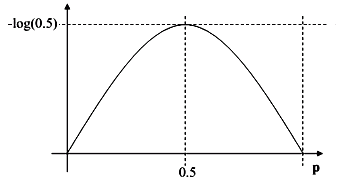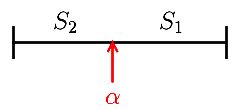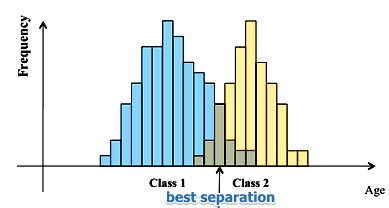# ML Wiki

## Information Gain

The expected information gain is

• the change in entropy when going
• from a prior state
• to anther new state

## Entropy

### Information

Consider two sequence of coin flips

• HHTHTTH...
• TTHHTTH...

How much information do we get after flipping each coin?

• information: $I(X) = - \log_2 p(X)$
• need a measure of unpredictability in a sequence of events
• Expected Value of information -
• $\text{Entropy}(X) = E[I(X)] = \sum_x p(x) \cdot I(x) = - \sum_x p(x) \cdot \log_2 p(x)$

E.g.

• coin: $P(H) = P(T) = 0.5$
• $I(X) = - \sum_i p(i) \log_2 p(i) = - (0.5 \log_2 0.5 + 0.5 \log_2 0.5) = 1$
• dice: $p(i) = 1/6$
• $I(X) = - \sum_i p(i) \log_2 p(i) = - 6 \cdot (\frac{1}{6} \log_2 \frac{1}{6}) \approx 2.58$
• more unpredictable than a coin
• weighted dice: $p(1) = ... = p(5) = 0.1$ and $p(6) = 0.5$
• $I(X) = - 5 \cdot (0.1 \log_2 0.1) - 0.5 \log_2 0.5 \approx 2.16$
• less unpredictable than a fair dice

### Entropy Function

Given $m$ classes, the entropy of signal $S$ is

• $I(S) = - \sum_{i=1}^{m} p_i \log_2 (p_i)$
• where $p_i$ is probability of seeing class $C_i$ in $S$
• $S_i$ - set of all records of class $i$

## Split in Halves

### Entropy for Two Classes

For two classes:

• If records in $S$ belong to classes $C_1$ or $C_2$
• Let $p_1 = ( |S_1| / |S| ) = p$ and $p_2 = ( |S_2 | / |S| ) = 1 - p$
• Then, $E[I(S)] = -p \cdot \log_2 (p) - (1 - p) \cdot \log_2 (1-p)$
• expected value of entropy with two subsets

Entropy is maximal when $p_1 = p_2 = 0.5$

• when $p_1 = 0$ and $p_2 = 1$ (or vise versa), there's no entropy### Splitting

Suppose we want to split a set $S$ into two parts $S_1$ and $S_2$

• we compute the entropy before splitting
• and compute the entropy after splitting at some point $\alpha$
• the information gain of splitting is the entropy before minus the expected entropy after

$I(S, \alpha) = I(S) - E[I(\{S_1, S_2\})] = I(S) - p_1 \cdot I(S_1) - p_2 \cdot I(S_2)$

• where $p_1 = \cfrac{|S_1|}{|S|}$ and $p_2 = \cfrac{|S_2|}{|S|}$
• and $S_1, S_2$ are subsets of $S$ s.t. $S_1 \equiv \{ s \ : \ s < \alpha \}$ and $S_2 = S - S_1$
• note that I(S) is a constant and doesn't depend on $\alpha$

### Best Splitting

What is the best $\alpha$:

• we want to select a boundary $\alpha$ that maximizes the information gainSo for all possible values of $\alpha$

• we compute the information gain
• and select the best splitting
• the process can be repeated recursively

### Example 1

• suppose that for the titanic dataset () we have 342 survived people out of 891
• $p(\text{Survive}) = \cfrac{342}{891}$
• \text{Entropy} = - \cfrac{342}{891} \log_2 \cfrac{342}{891} - \cfrac{549}{891} \log_2 \cfrac{549}{891} \approx 0.96

This is important measure for splitting in Decision Trees

• select the attribute with the highest information gain
• it will reduce the unpredictability the most

### Example 2

Suppose we have a data set of customers

• class $C_1$ - drinks milk and $C_2$ - doesn't drink
• what's the best boundary to split the age variable?
•